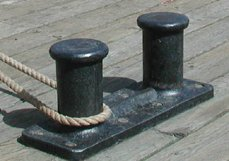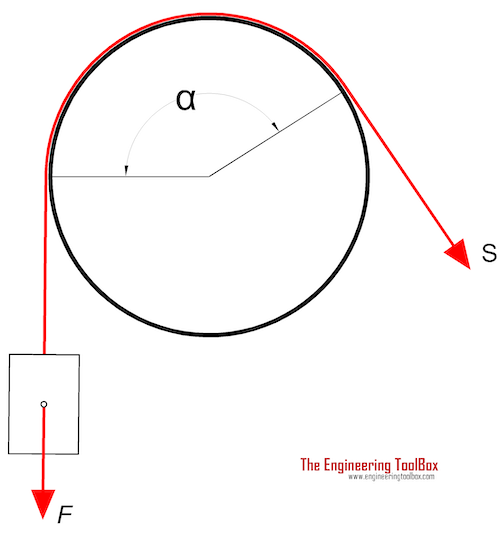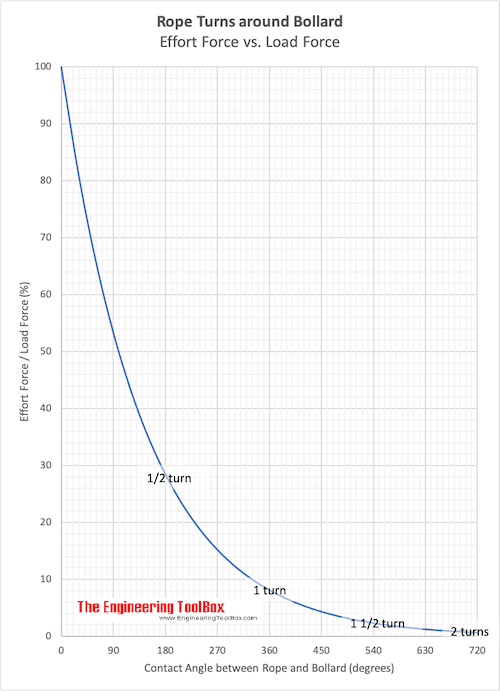Engineering ToolBox - Resources, Tools and Basic Information for Engineering and Design of Technical Applications!

# Bollard Forces

## Friction, load and effort forces acting in ropes turned around bollards.Bollards are common on quays and used to moore ships and boats. By turning a rope around a pole the effort force required to hold the load force can be reduced dramatically.The effort force in a rope around a bollard can be calculated as

S = F e-(μ αrad)                         (1)

where

S = effort force in the rope (N, lb)

F = load force (N, lbf)

e = 2.718..

μ = friction coefficient (approximately 0.3 - 0.5 is common for a rope around a steel or cast iron bollard)

αrad = 2 π αdegrees / 360 = angle where the rope is in contact with the bollard (radians

αdegrees = angle where the rope is in contact with the bollard (degrees)

### Angle - turns, degrees and radians

• 1/4 turn : 90 degrees => α = 1/2 π
• 1/2 turn : 180 degrees => α = π
• 1 turn : 360 degrees => α = 2 π
• 2 turns : 720 degrees => α = 4 π

### Example - A rope with One turn around a Bollard

One turn equals to 360 degrees or 2 π radians. With a friction coefficient of 0.5 the effort force in the rope can be calculated as

S = F e-(0.5 2 π)

= 0.043 F (N)

One turn around the bollard reduces the required effort force to less than 5% of the load force.

### Example - Shoring a ship

The retardation (negative acceleration) of a ship arriving at quay with velocity 0.05 m/s and stopped within 2 seconds - can be calculated as

a = dv/dt                                (2)

= (0.05 m/s) / (2 s)

= 0.025 (m/s2)

With ship mass 20000 kg the required force in the rope from the ship (load) can be calculated as

F = m a                                      (3)

= (20000 kg) (0.025 m/s2)

= 500 N

= 0.5 kN

The required effort force in the rope with a half turn around the bollard (180 degrees or π radians) with a friction coefficient of 0.4 can be calculated as

S = (500 N) e-0.4 π

= 142 N

= 0.14 kN

The Effort Force - Load Force ratio for various rope angles are indicated in the chart below:• friction coefficient 0.5

### Bollard (or Pole) Effort Force Calculator

This calculator is based on the equation above and can be used to calculate the effort force in ropes turned around poles or bollards. Note that the calculator uses degrees for the turns.

friction coefficient

angle where the rope is in contact with the pole (degrees)

## Related Topics

• Dynamics - Motion - velocity and acceleration, forces and torque.
• Mechanics - Forces, acceleration, displacement, vectors, motion, momentum, energy of objects and more.
• Statics - Loads - forces and torque, beams and columns.

## Engineering ToolBox - SketchUp Extension - Online 3D modeling!

Add standard and customized parametric components - like flange beams, lumbers, piping, stairs and more - to your Sketchup model with the Engineering ToolBox - SketchUp Extension - enabled for use with the amazing, fun and free SketchUp Make and SketchUp Pro .Add the Engineering ToolBox extension to your SketchUp from the SketchUp Pro Sketchup Extension Warehouse!

Translate

## Privacy

We don't collect information from our users. Only emails and answers are saved in our archive. Cookies are only used in the browser to improve user experience.

Some of our calculators and applications let you save application data to your local computer. These applications will - due to browser restrictions - send data between your browser and our server. We don't save this data.

## Citation

• Engineering ToolBox, (2008). Bollard Forces. [online] Available at: https://www.engineeringtoolbox.com/bollard-force-d_1296.html [Accessed Day Mo. Year].

Modify access date.

. .

#### Scientific Online Calculator6 24